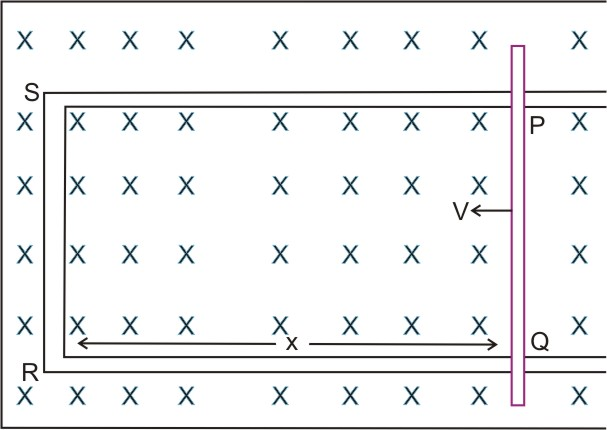# (a) State Faraday's law of electromagnetic induction.(b) Derive expression for the emf induced across the ends of a straight conductor of length l moving right angles to uniform magnetic field B with a uniform speed v.(c) Obtain expression for the magnetic energy stored in a solenoid in terms of the magnetic field B, area A and length l of the solenoid through which a current i is passed.

(a)

Faraday's first law of electromagnetic induction:

Whenever a conductor is placed in a changing magnetic field, an electromagnetic force is induced in it (which is called as induced emf). If the conductor is closed the current is induced in it, which is called induced current.

Faraday's second law of electromagnetic induction:

The magnitude of induced electromotive force in a circuit is equal to the rate of change of magnetic flux linked with the coil.

Mathematically induced emf is,

(b)Here the length of the conductor is 'P' which is moving right angle to the magnetic field 'B' with the uniform speed v.

In the figure, the conductor PQ is free to move.

Consider the rectangular loop PQRS. The magnetic flux through the loop is Blx.

That is  where x is length of RQ

When the conductor moves x is varying. So due to the rate of change of magnetic flux an emf is induced in it.

According to Faraday's law of electromagnetic induction

This induced emf Blv is motional emf.

(c) We know that

Induced emf                  .......(1)

where L = self inductance

I = current

The rate of work needed in an electric circuit is

............(2)

Substituting (1) in (2)  we get

we know that

By integrating we get

For a solenoid of  area A and length l, the magnetic field due to the current flowing through is

Total flux

Self-inductance

Therefore energy stored

### Preparation Products

##### Knockout NEET July 2020

An exhaustive E-learning program for the complete preparation of NEET..

₹ 15999/- ₹ 6999/-
##### Rank Booster NEET 2020

This course will help student to be better prepared and study in the right direction for NEET..

₹ 9999/- ₹ 4999/-
##### Knockout JEE Main July 2020

An exhaustive E-learning program for the complete preparation of JEE Main..

₹ 12999/- ₹ 6999/-
##### Test Series NEET July 2020

Take chapter-wise, subject-wise and Complete syllabus mock tests and get in depth analysis of your test..

₹ 4999/- ₹ 2999/-